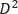# How to Calculate Steel Quantity for Slab, Footing and Column?

Estimation of steel reinforcement quantity for concrete slab, footing and column, beams etc. is crucial for the cost evaluation for the construction. Design drawings are used as a base for computing rebar quantity in different structural elements.

This article presents steel quantity computation process for slabs, columns, and footings.

## Calculate Steel Quantity for Slab

1. Obtain slab dimension and reinforcement details from design drawings as shown in Fig.1.
2. Compute number of steel bars.

Main Steel Bars

No. of bars= (Slab length(L)/spacing)+1               Equation 1

Shrinkage and Temperature Steel Bars

No. of bars= (Slab length(S)/spacing)+1               Equation 2

In equation 1, center to center spacing of main reinforcement steel bars are used and shrinkage and temperature bar spacing is used in equation 2.Fig. 1: Types and arrangement of steel bars in one way slab

3. Calculate cutting length:

Main steel bars

Cutting length= clear span(S)+Ld+inclined length+2×45 degree bend      Equation 3

Shrinkage and Temperature steel bars

Cutting length= clear span(S)+Ld+inclined length+2×45 degree bend      Equation 4

Where:

Ld: development length which illustrated in Fig. 2.

Inclined length can found from the following expression:

Inclined length= 0.45D                                                    Equation 5

D=slab thickness-2*concrete cover-bar diameter               Equation 6Fig. 2: Bent up bars in slab

3. Convert that length into kilograms or tons because steel bars are ordered by weight. The same equation used for both main and shrinkage and temperature reinforcement, but corresponding cutting length, number of bars, and bar diameter is used.

Main steel bars=No. of bars*cutting length*weight of the bar (/162)     Equation 7

(/162) is the weight of a steel which is derived from steel volume times its density which is 7850 kg/m3.

## Calculate Steel for Footing

Size of footing and its reinforcement details (bar size and spacing) shall be known. This can be achieved from design drawings. After that, the following steps will be taken to compute steel quantity.

1. calculate the required number of bars for both directions.

No. of bars = {(L or w – concrete cover for both sides) ÷ spacing} +1       Equation 8

where L or W: length or width of footing

1. Then, find the length of one bar

Length of bar = L or W–concrete cover for both sides + 2*bend length     Equation 9

Where L or W is length or width of footing

1. After that, compute the total length of bars which is equal to the number of required bars multiply by the length of one bar. If the same size of bars is used in both directions then you can sum up both quantity of the bars
2. Convert that length into kilograms or Tons. This can be done by multiplying cross section area of steel by its total length by density of steel which 7850 kg/m3

The above calculation procedure is for  single reinforcing net. Therefore, for footings with the double reinforcing net, the same procedure need to be used again to compute steel quantity for another reinforcing net.

## Calculate Steel Quantity for Columns

Achieve column size and reinforcement detailing from design drawings. Then, compute quantity of steel in the column using the following steps:

### Longitudinal steels

1. Compute total length of longitudinal bars which equal to the column height plus laps for footing multiply number of longitudinal bars.
2. Convert that length into kilograms or Tons. This can be done by multiplying cross section area of steel by its total length by density of steel which 7850 kg/m3

### Stirrups

1. Compute cutting length of stirrups using the following equation

Cutting length=2*((w-cover)+(h-cover))+Ld                             Equation 10

where:

w: column width

h: column depth

Ld: stirrup development length

2. Calculate number of stirrup by dividing column height over stirrup spacing plus one.

3. Estimate total length of stirrup which is equal to stirrup cutting length times number of stirrups.

4. Convert that length into kilograms or Tons. This can be done by multiplying cross section area of steel by its total length by density of steel which 7850 kg/m3.

Total steel quantity of column equal to the sum of both main and stirrup steels.